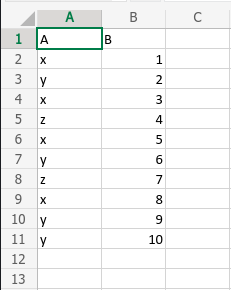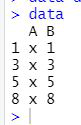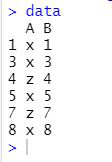Related Articles

# Subset Dataframe Rows Based On Factor Levels in R

• Last Updated : 23 Sep, 2021

In this article, we will be discussing how to subset a given dataframe rows based on different factor levels with the help of some operators in the R programming language.

## Method 1: Subset dataframe Rows Based On One Factor Levels

In this approach to subset dataframe rows based on one-factor levels, the user just needs the in-built operator of the R programming language, for this, the user needs to use the \$ and == operators with the data and provide the required details into it specifically to retain the rows where our factor column has one specific factor level in the R programming language.

File Used:Example: Subset dataframe rows based on one-factor levels

## R

 `data=``read.csv``(``'data_gfg.csv'``)``data=data[data\$A == ``"x"``, ]``data`

Output:## Method 2: Subset dataframe Rows Based On Multiple Factor Levels

In this approach to subset the dataframe rows based on the multiple factor levels, the user simply needs to use the in-built operators of the R programming language, which will be including \$,% and a vector which will be containing the required multiple factor levels, as per the requirements of the user to retain the rows where our factor column has multiple specific factor level in the R programming language.

Example: Subset dataframe rows based on multiple factor levels

## R

 `data=``read.csv``(``'data_gfg.csv'``)``data=data[data\$A %``in``% ``c``(``"x"``, ``"z"``), ] ``data`

Output:My Personal Notes arrow_drop_up